Browse by Topic
Related Topics

# Learning Differential Equations in 10 Hours or Fewer with the Wolfram Language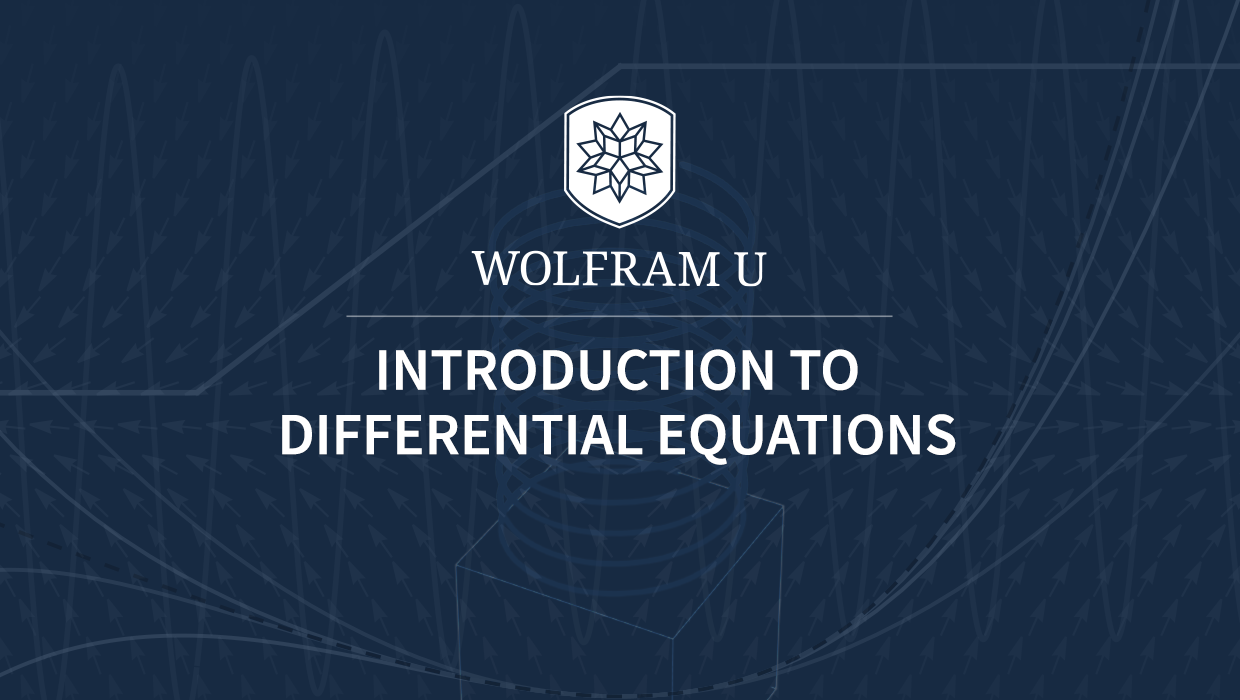Differential equations are a cornerstone of modern mathematics. From quantum mechanics to population dynamics and stock market predictions, they play a crucial role in understanding the world around us. For this reason, courses on differential equations are core for many undergraduate degrees in the natural sciences, engineering and other fields.

Today, I am proud to announce a free interactive course, Introduction to Differential Equations, that will help students all over the world learn and master this difficult but tremendously useful branch of mathematics. The course will investigate the methods to solve a wide class of differential equations while making extensive use of the powerful functions to solve them in the Wolfram Language.

Want to get started? Explore the interactive course by clicking the following image before reading the rest of this blog post.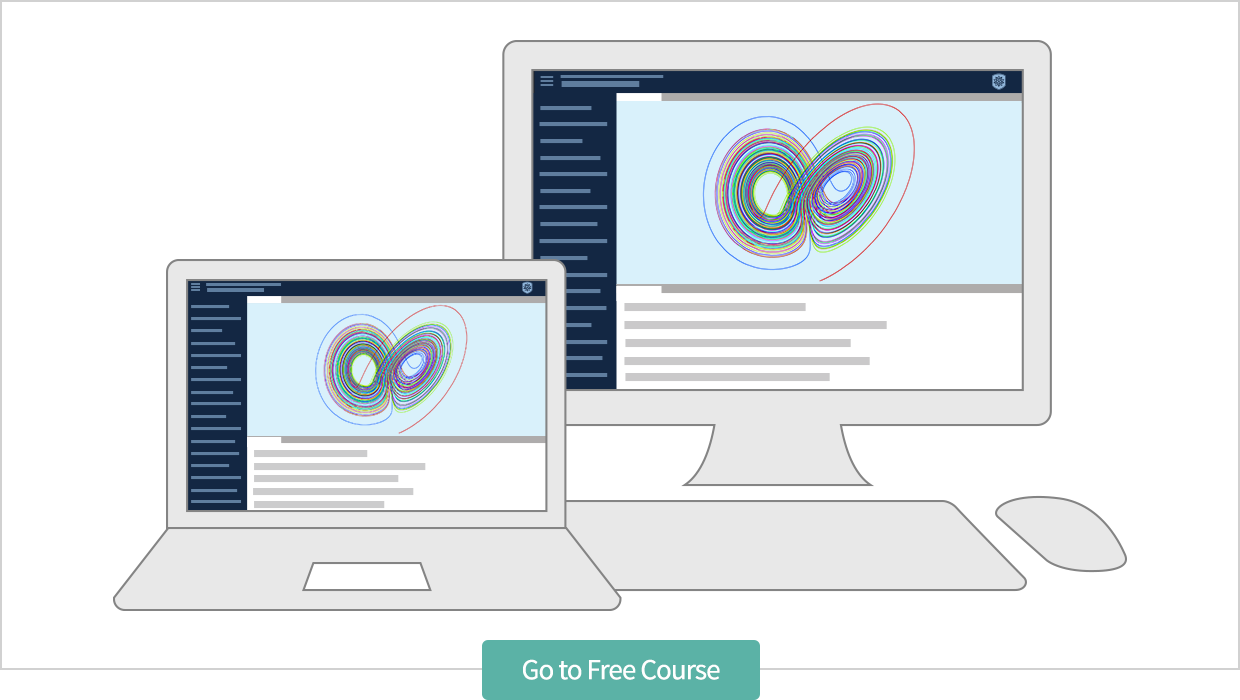## Motivation from History

Many of the great mathematicians in history have contributed to the development of the subject of differential equations. This began with Isaac Newton and Gottfried Wilhelm Leibniz and their introduction of the derivative in the seventeenth century. With the concept of the derivative of a function established, mathematicians could begin investigating equations involving functions and their derivatives. Such equations are known today as differential equations.

The study of differential equations brought about significant advancements in the eighteenth and nineteenth centuries. The work of Leonhard Euler formally established the solution methods for many types of differential equations during this time. Later, Pierre-Simon Laplace introduced his powerful integral transform, which can be applied to a wide range of differential equations. These methods of Euler, Laplace and others will be discussed throughout this course.

## Overview

Students taking this course will receive a thorough introduction to differential equations. This includes standard topics like first- and second-order differential equations and initial value problems. Introduction to Differential Equations will also discuss some more advanced topics like special functions, the Laplace transform and systems of differential equations.

Here’s a sneak peek at some of the course topics (shown in the left-hand column):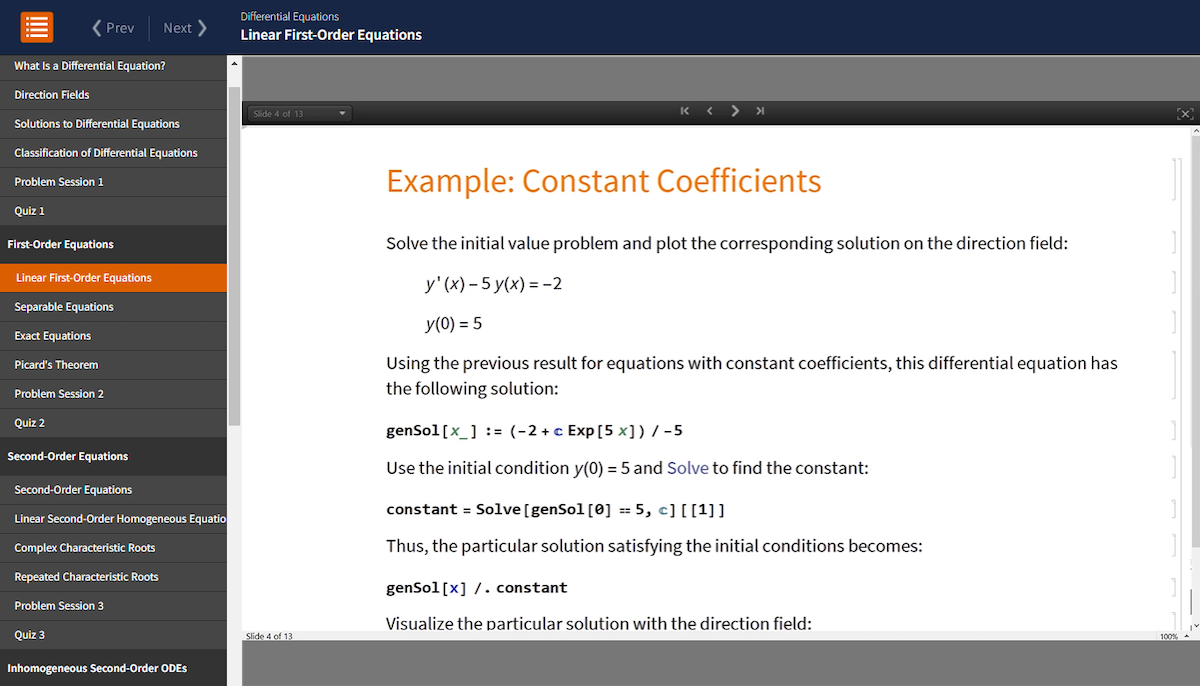I worked diligently to keep the course at a manageable length, and you will be able to finish watching all 31 videos and complete the eight short quizzes in about 10 hours.

Finally, I assume that students are familiar with college-level algebra, trigonometry and basic calculus. A background in linear algebra will be useful but not necessary, as any concepts from linear algebra that are needed will be reviewed in this course.

The next few sections of this blog post describe the different course components in greater detail.

## Lessons

The backbone of the course is a set of 31 lessons, beginning with “What Is a Differential Equation?”. This introductory lesson—see an overview in the following video—includes a discussion about the applications of differential equations. This is followed by a brief history of differential equations and a discussion about where differential equations arise in the modern world, and concludes with an outline of the course.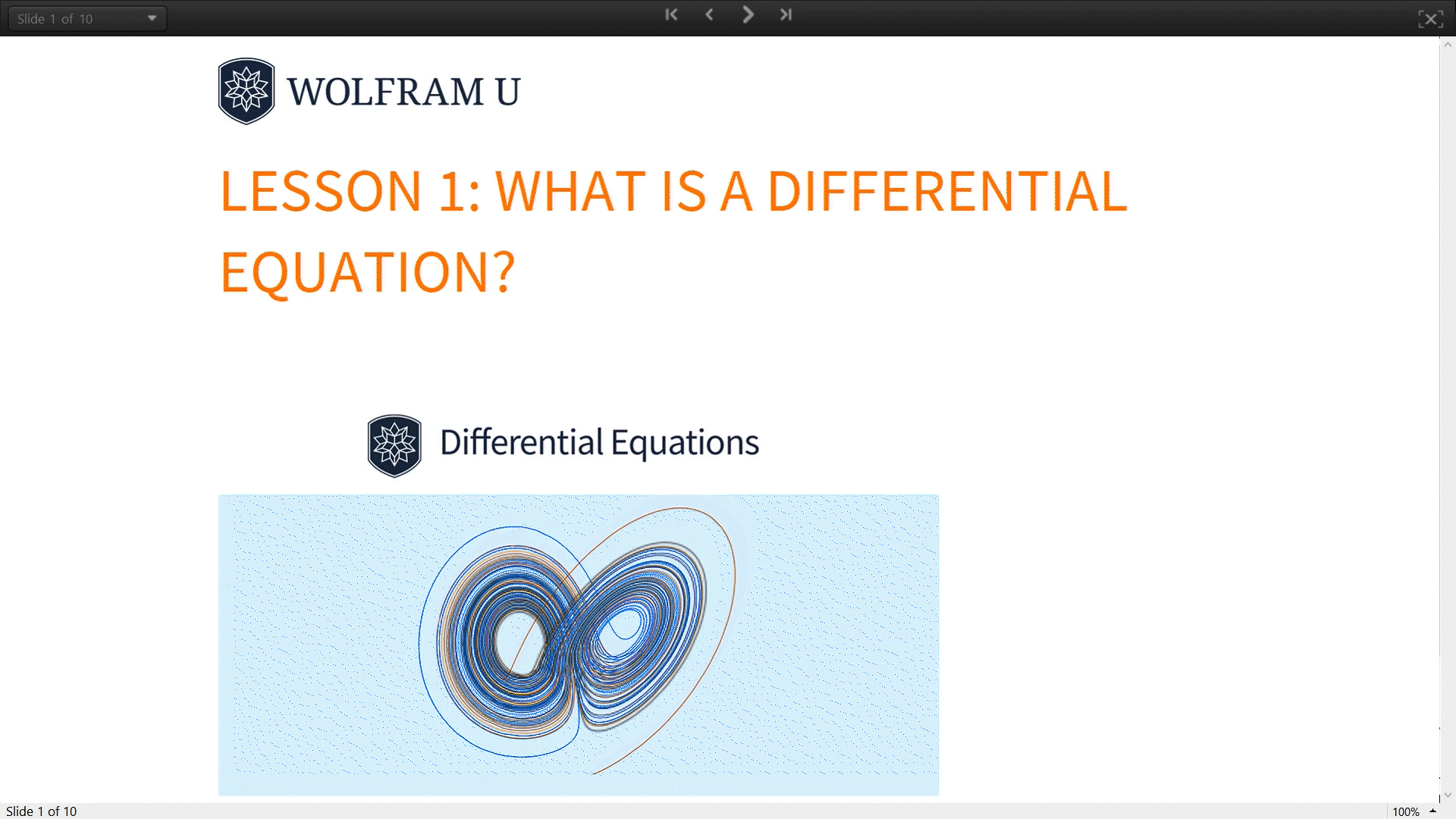Other lessons begin with a topic overview, such as direction fields or power series solutions, followed by a discussion of the key concepts with examples interspersed that illustrate the main ideas using Wolfram Language functions to verify solutions or visualize results.

The videos range from 10 to 15 minutes in length, and each video is accompanied by a transcript notebook displayed on the right-hand side of the screen. Copy and paste Wolfram Language input directly from the transcript notebook to the embedded scratch notebook to try the examples for yourself.

## Exercises

Each lesson includes a set of five exercises to review the concepts covered in it. This course is designed for independent study, so detailed solutions are also provided for all exercises, as per this example: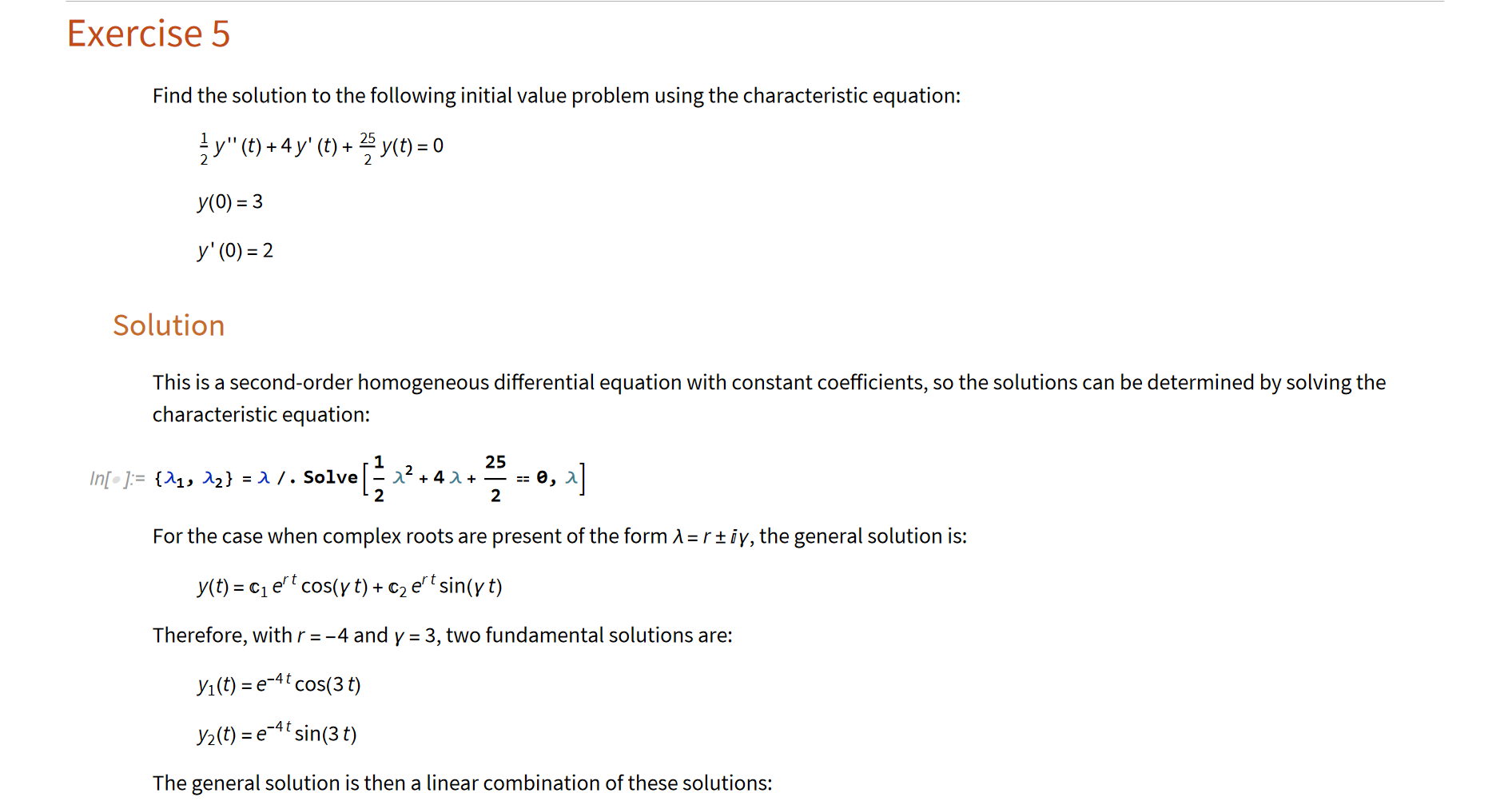The notebooks with the exercises are interactive, so students can try variations of each problem in the Wolfram Cloud. In particular, they are encouraged to change the coefficients of the differential equations, add terms and experience the awesome power of the Wolfram Language for solving differential equations.

## Problem Sessions

Each section of the course includes a problem session that solves in detail several example problems that are related to the ideas developed in the section. These problem sessions are meant to reinforce the material that has been learned so students can solidify their understanding.

The following is an excerpt of the video for Problem Session 1: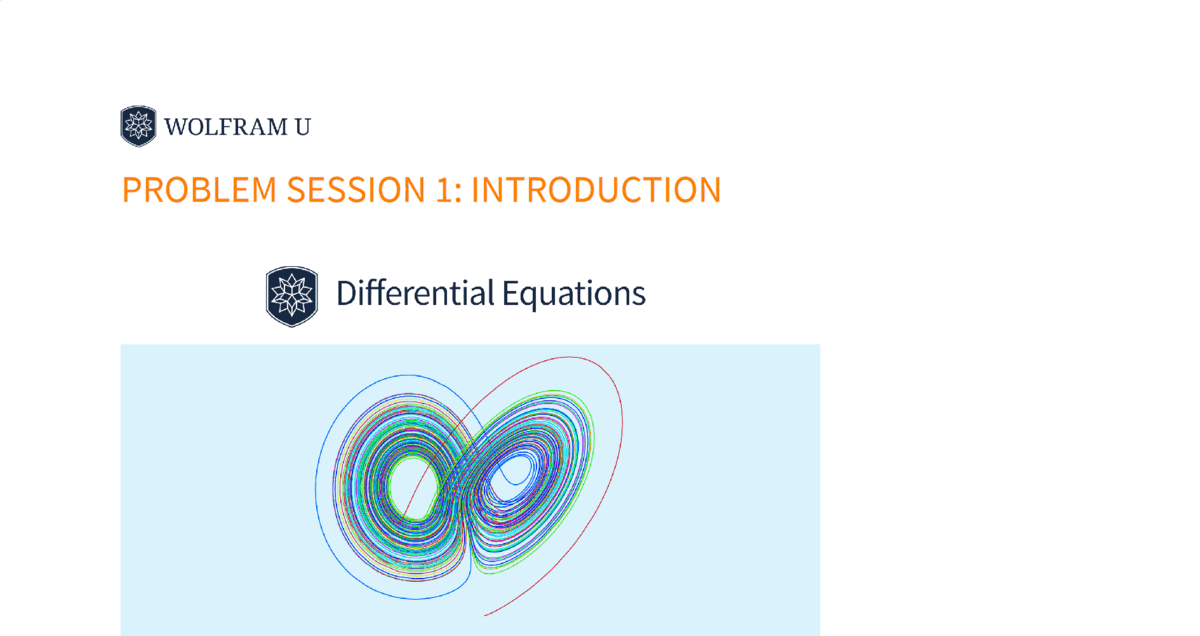## Quizzes

Each course section concludes with a short, multiple-choice quiz with five problems. The quiz problems are at roughly the same level as those covered in the lessons, and a student who reviews the section thoroughly should have no difficulty in doing well on the quiz.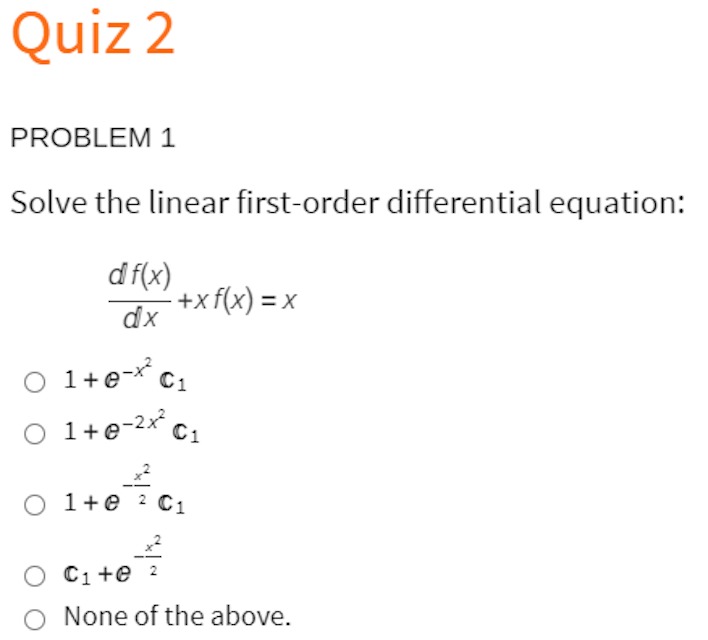Students receive instant feedback about their quiz question responses, and they are encouraged to try any method—including hand or computer calculations—to solve them.

## Course Certificate

Students should watch all the lessons and problem sessions and attempt the quizzes in the recommended sequence because course topics often rely on earlier concepts and techniques. At the end of the course, you can request a certificate of completion. A course certificate is earned after watching all the lessons and passing all the quizzes. It represents proficiency in the fundamentals of differential equations and adds value to your resume or social media profile.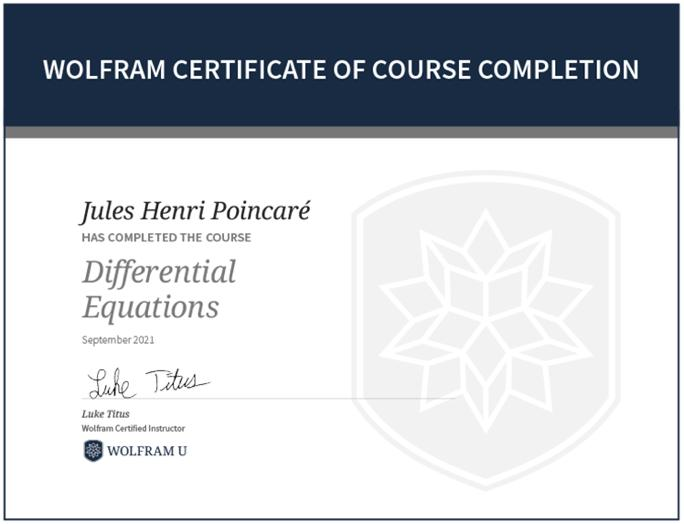An optional final exam is also available at the end of the course. Passing the exam allows you to receive an advanced level of certification.

## A Building Block for Success

Mastering the fundamental concepts of differential equations is integral for students in the fields of science, technology, engineering and math (STEM) and is an immense intellectual achievement for anyone in any field. I hope that Introduction to Differential Equations will help you to achieve this mastery and contribute to your success in your chosen field. I enjoyed creating and teaching this course and welcome any comments regarding the current course as well as suggestions for future courses.

## Acknowledgements

I would like to thank Devendra Kapadia, Cassidy Hinkle, Aaron Crenshaw, Amruta Behera, Joyce Tracewell, Veronica Mullen and Bob Owens for their dedicated work on various aspects (lessons, exercises and videos) of the course.

 Want more help? Register for one of Wolfram U’s Daily Study Groups.